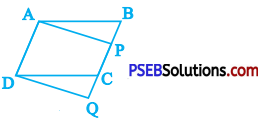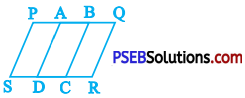# PSEB 9th Class Maths Solutions Chapter 9 Areas of Parallelograms and Triangles Ex 9.1

Punjab State Board PSEB 9th Class Maths Book Solutions Chapter 9 Areas of Parallelograms and Triangles Ex 9.1 Textbook Exercise Questions and Answers.

## PSEB Solutions for Class 9 Maths Chapter 9 Areas of Parallelograms and Triangles Ex 9.1

Question 1.
Which of the following figures lie on the same base and between the same parallels. In such a case, write the common base and the two parallels.

(i)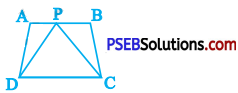In figure (i), trapezium ABCD and ∆ PDC lie on the same base and between the same parallels.
Here, DC is the common base and DC and AB are two parallels.(ii)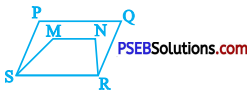In figure (ii), no two figures lie on the same base and between the same parallels.

(iii)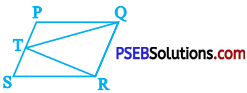In figure (iii), parallelogram PQRS and ∆ TQR lie on the same base and between the same parallels.
Here, QR is the common base and QR and PS are two parallels.(iv)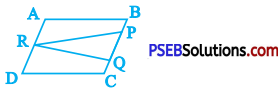In figure (iv), no two figures lie on the same base and between the same parallels.

(v)